## Bars are boring! Use cute little shapes instead!

Today’s topic might not necessarily be best practice in terms of visualisation, but scores really high on the prettiness / quirkiness scale. I will show you how to replace your bars with… other bars, except that they will be made of little shapes 🙂 After we’ll be done you will have your own variation of the image below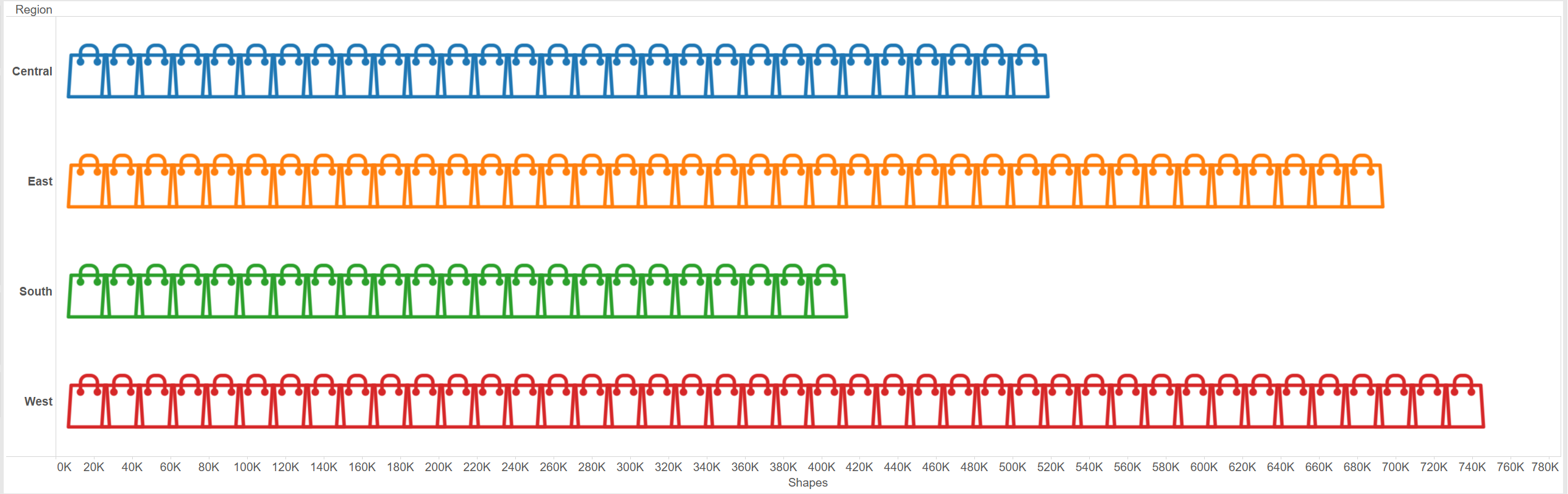Start by creating the most standard, boring, bar chart visualisation you can think of. I have used, unsurprisingly, Sales by Region from the Superstore Dataset. Then change the mark type from bar to shape. Next, create an Index field, that contains only the Index formula.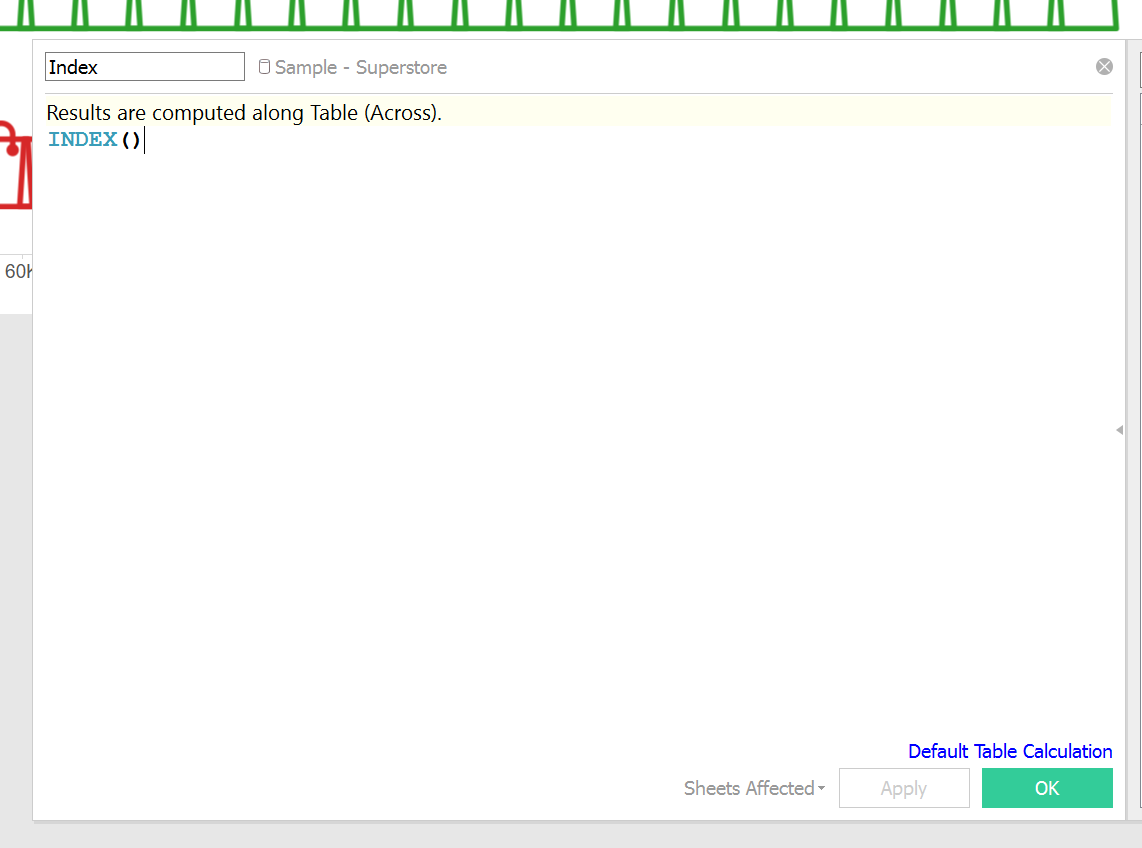The Index field is the little trick that Tableau will use to count our data, slice it and show many little shapes in place of a continuous bar. For now don’t worry about it, go find the shape you want to use and add it in its own folder in the Shapes folder within the Tableau folder in your Documents. Now reload your shapes from the shapes mark card and assign your custom shape in place of the default one.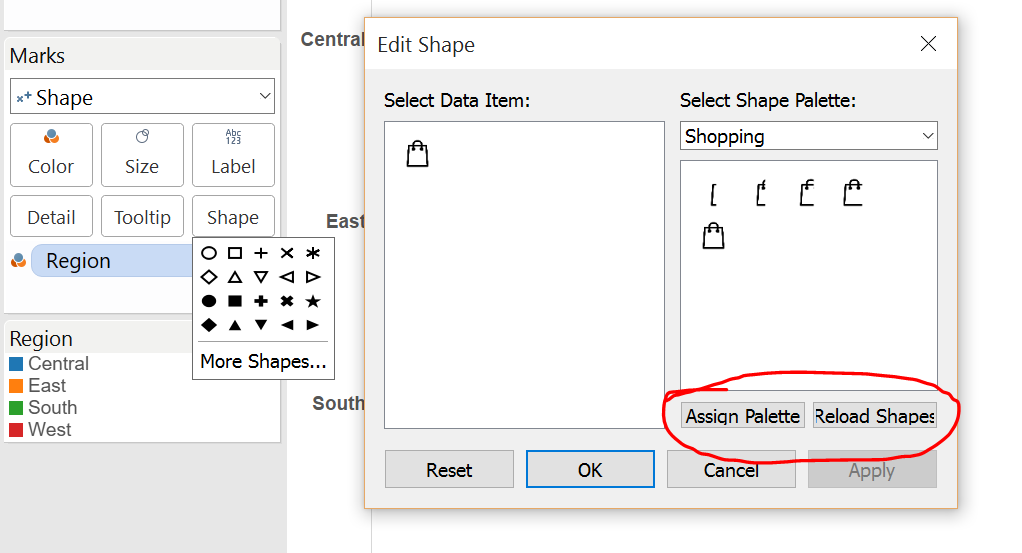Your shape should have replaced the standard Tableau circle and your visualisation should look something like this.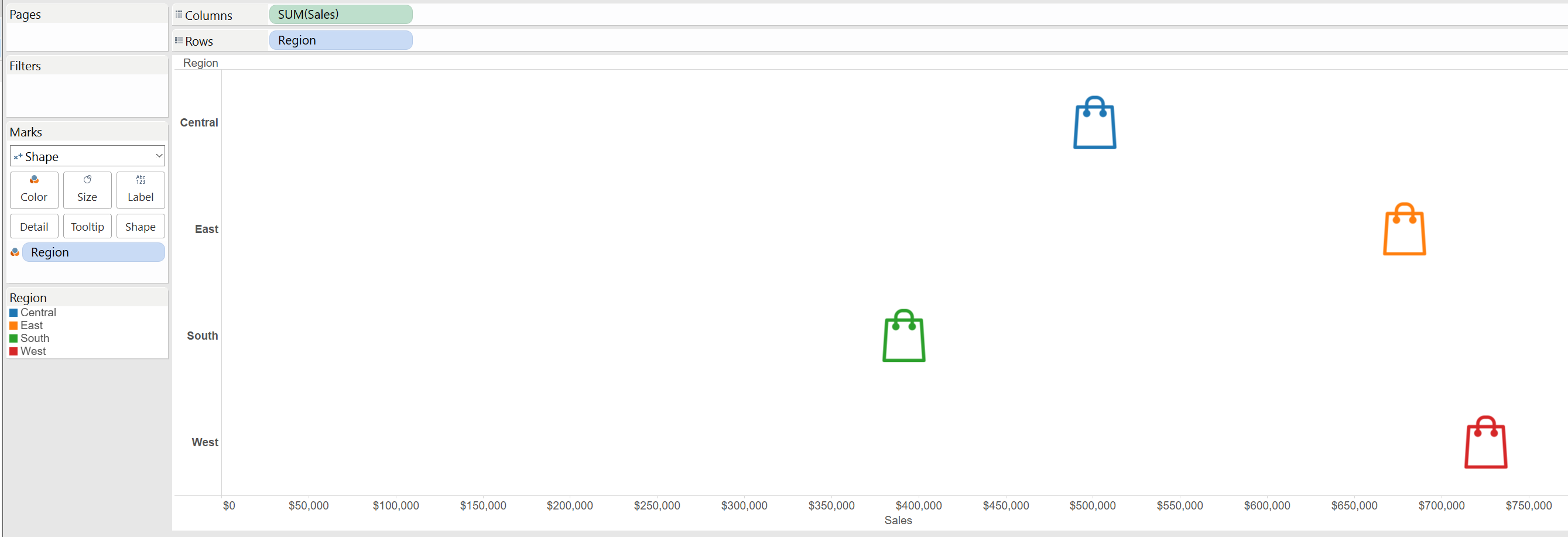We need two more calculated field. The formula looks a bit complicated, but what you need to take away from it is that it is dividing your measure by some arbitrary number (you will have to figure this out yourself, but I will return to this later) and using the index function multiplied by the same arbitrary number to create chunks, each of which will be represented by one shape. The formula you need to use is the following

IF INDEX()-1 <= INT(WINDOW_SUM(SUM([Sales]))/17500)
THEN
INDEX()*17500
END

Now replace the measure in your view with this calculated field, make sure it is computed by cell and that the Aggregate Measure in the Analysis menu is unticked and this is what you should have at this stage.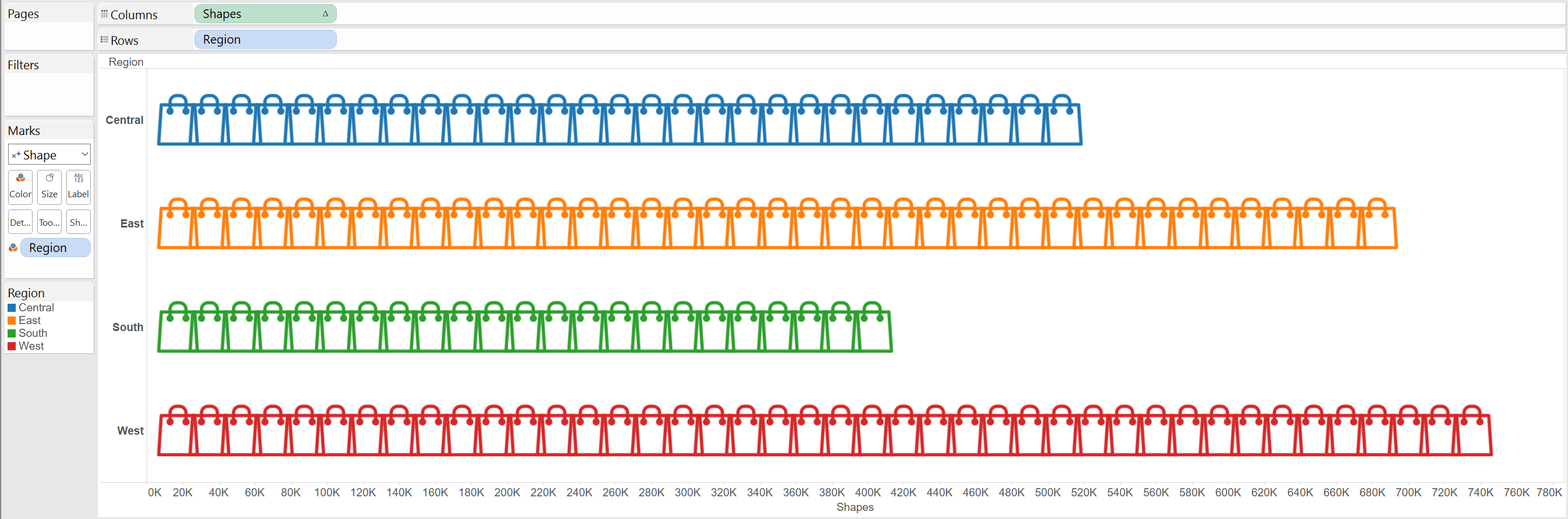If this is all you want you can stop here. I have proceeded to create a formula that will allow me to see the running total for each shape which can be added to the tooltip to provide more information to the user. It’s almost the same formula as before:

IF [Index] <= INT(WINDOW_SUM(SUM(Sales))/17500) THEN
INDEX()*17500
ELSEIF [Index]-1 <= INT(WINDOW_SUM(SUM(Sales))/17500) THEN
WINDOW_SUM(SUM(Sales))
END

At this point you might need a bit more precision and to show different shapes for smaller chunks of your data. Another calculated field can help us telling Tableau to look at the last portion of the data that cannot be sliced evenly and according to what this amount is to divide it in four categories to which a custom shape can be added. Here is the formula:

IF [Index] <= WINDOW_SUM(SUM([Product Base Margin]))/150
THEN
“80 to 100”
ELSE
CASE INT(((WINDOW_SUM(SUM([Product Base Margin]))/150) – [Index]+1)*0.15)
WHEN 0 THEN “<20”
WHEN 1 THEN “20 to 40”
WHEN 2 THEN “40 to 60”
WHEN 3 THEN “60 to 80”
END
END

I have used this formula in another dataset which had smaller values and for which more precision was required. Here is the result: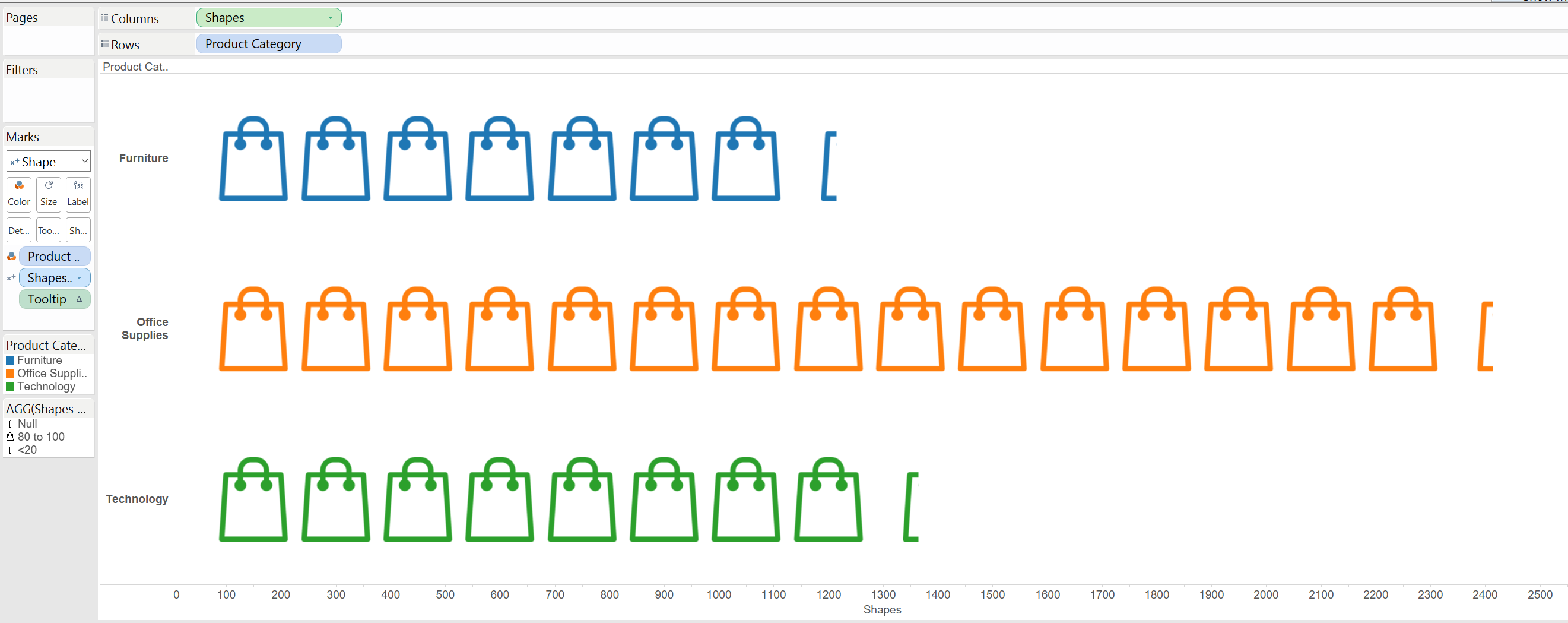The small shape you see at the end is a (poorly) cropped shape that tells the user that the last chunk of data is equal to less than 20% of a full chunk. That is almost everything for this topic, there are two things you should pay attention to:

• The numbers by which you are dividing your dimension, it’s a bit of a trial and error. If you want your shapes to be closer choose smaller numbers, if you want them to be more far apart choose larger numbers. I have used 17500 for the superstore example and 150 for the second example. Needless to say, higher numbers in your measure also mean you should divide by a larger number.
• Use png for your shapes. They are transparent, so won’t look ugly if your background is not white. Also, I couldn’t get JPEGs to work, they would show up as filled rectangles rather than shapes. On the other hand, it could be my computer playing some funky trick on me

So, this should be enough to get you started with bars and shapes. If you want to experiment with even more crazy stuff I strongly recommend this blog post: http://www.google.com/url?q=http%3A%2F%2Ft.co%2F4GsvE9ypGi&sa=D&sntz=1&usg=AFQjCNHObbzD3aOosarNff8HnAbj_bjv4Q